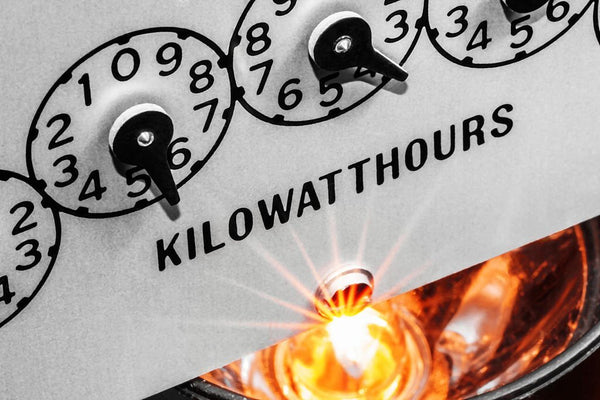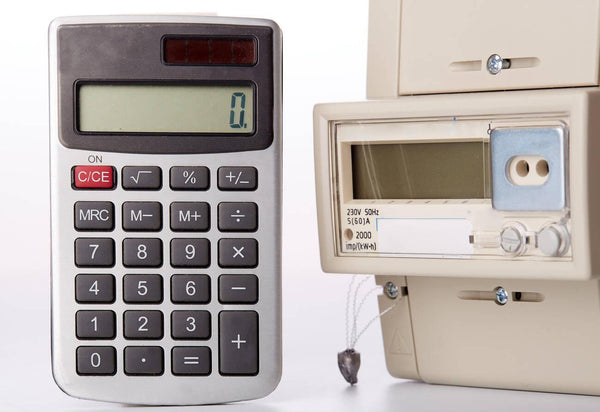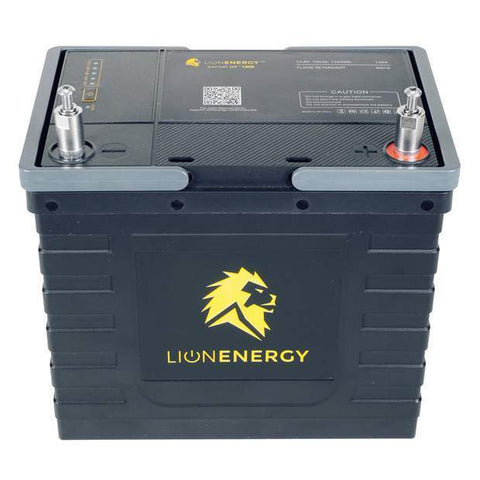Househoule Watt Calculator: Appliances and Tools - ShopSolar.com

# Household Watt Calculator

To understand the amount of electricity that you consume, you need to understand the wattage of your appliances and how much energy they consume.

That’s where our handy watt calculator comes in. It allows you to understand the wattage of your appliances and how this translates into electricity usage.

## What Is Wattage?

A watt is a unit of power that is used to measure the rate of electricity transfer. This tells you how much an appliance uses when it is running.There are also different ways of representing wattage, and other important units of electricity. You might need to convert VA to watts, or kilovolt amperes (kVA) to watts.

In a DC system, the general rule is that 1W is equal to 1VA, but you can get more precise calculations.

Different items around your house have different wattages. For example, a standard incandescent light bulb will be around 60 watts. However, an equivalent LED light bulb will only be around 10 watts.

Understanding the wattage of various appliances allows you to understand where you can cut costs when it comes to your electricity bill.

## Household Watt Calculator

To work out the number of watts your household uses, you need to look at the wattage of various devices. Most time, the wattage of an appliance will be listed on the bottom.

However, sometimes your device will only tell you the voltage, but luckily you can convert volts to watts. Most appliances use 120 volts, and you can work out the wattage using this information.

The average U.S. household uses around 11,000 watts of electricity per year. With a household watt calculator, you can get a more precise understanding of your actual consumption.

If you add up the wattage of the appliances in your home and then estimate the number of hours that you use each of them, you can multiply the watts by the hours to get the watt-hours.

You can also do this for individual appliances. The basic household watt calculation takes the wattage of an appliance, times the number of hours you are using it, and divided by 1,000.

This tells you your energy consumption in kilowatt-hours (kWh). Once you know this, you know your energy consumption in a day, which you can use to extrapolate your usage in a month or a year.To come back to the light bulb example, if your 60-watt incandescent bulb is on for around 6 hours each day, you can multiply the wattage by the number of active hours. This gives you 600 watt-hours (Wh). You can then divide this by 100 to get the kilowatt hours (kWh) of 0.6kWh.

Comparing this to a 10-watt LED light bulb, it would only consume 0.1 kWh per day, making for a massive saving in both electricity and cost.

## Why Is a Household Watt Calculator Useful?

Once you’ve used the household watt calculator, you can use the cost of a watt-hour to work out how much you are spending on electricity in a year, or a month.

It is important to remember that a household watt calculator does not include taxes, and you would have to add these on afterward to fully understand the cost of electricity in your house.

By using a household watt calculator, you can understand which appliances are using the most electricity and use this information to reduce your electricity bill. This can be good for both the environment and your pocket.

Another option is to use a PV watts calculator. This is a good way of understanding the wattage of a photovoltaic (PV) system that is connected to the grid.

### Solar Power

Another way to reduce your electricity costs is to install solar panels to help reduce your reliance on the grid. While a solar system can be a large upfront investment, it can save you money in the long run.

You can use a household watt calculator to work out your energy consumption to help you choose what kind of solar system will meet your needs.

To use solar power to offset some of your electricity costs, you can look at how much a solar panel can produce, and work out how much energy you want to produce using a solar system.The household watt calculator can also help you choose a deep-cycle solar battery that will store the energy produced by your solar panels.

You might also need to convert milliamp hours (mAh) to watt hours (Wh). An ampere is the amount of power that a battery can produce in one hour.

Did You Find Our Blog Helpful? Then Consider Checking: